## C# Aircraft Instrument Control

1

The aim of this C# project is to purpose six aircraft cockpit instruments usable in forms as any other C# controls and to define a generic instrument class in order to design any kind of dashboard instruments.

## 2

The controls are built with bitmaps which are rotated, translated or scaled before to be displayed. The basic methods for rotate, translate and scale images are defined in the mother class. Each control then uses its dedicated parameters (related to a physical signification) in order to manipulates the images.

## Aircraft Instruments

• Air speed indicator: airspeed (kts)
• Attitude Indicator: pitch (deg), roll (deg)
• Altimeter: altitude (ft)
• Turn Coordinator: turn rate (deg/min)
• Vertical speed indicator: vertical speed (ft/min)

## 3

This section explains in detail the implementation of the basic functions defined in the ``` Instrument Control ``` class.

### Rotate Image

#### Implementation

The rotation of the image is divided in two main parts:

First, the rotation of the
``` PaintEventArgs ```coordinate system around the upper left corner of the drawing area.

Second, the drawing of the image corrected by translation offset in order to display the image as if it has turned around a user defined point.Let’s see step by step:

Step 0: Initial situation.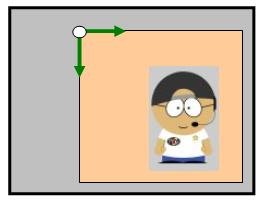Step 1: Rotate the ``` PaintEventArgs ``` coordinate system around the left upper corner of the paint area.Corresponding code sample:

```// Rotate image support
pe.Graphics.RotateTransform((float)(alpha * 180 / Math.PI));```

Step 2: Draw the image and apply the translation correction.Corresponding code sample:

```// Display image
pe.Graphics.DrawImage(img, (ptImg.X + deltaX) * scaleFactor, (ptImg.Y + deltaY) *
scaleFactor, img.Width * scaleFactor, img.Height * scaleFactor);```

Step 3 (Final step): Put the ``` PainEventArgs ```coordinate system as found.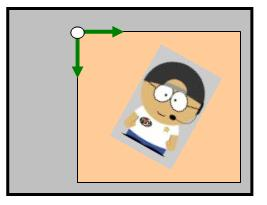Corresponding code sample:

```// Put image support as found
pe.Graphics.RotateTransform((float)(-alpha * 180 / Math.PI));```

The key point in those operations is the calculation of the translation correction coefficients.

The next figure explains the geometrics considerations:G0 is the user defined rotation center
G1 is the G0 position after the step 1.

The aim of this section is to identify the G1G0 translation and apply the corresponding offset in order to draw the rotation point as if it has not moved.

Then we work with the geometrics definitions:

 a.b.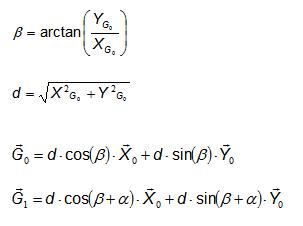c.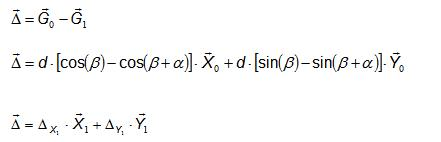d.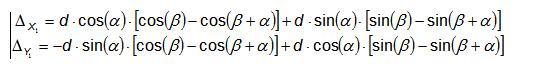As a result, the offset coefficients are: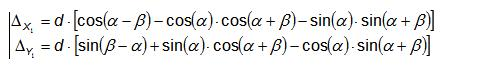The corresponding code sample is as follows:

```// Computed offset
deltaX = (float)(d * (Math.Cos(alpha - beta) - Math.Cos(alpha)*
Math.Cos(alpha + beta) - Math.Sin(alpha) * Math.Sin(alpha+ beta)));
deltaY = (float)(d * (Math.Sin(beta - alpha) + Math.Sin(alpha)*
Math.Cos(alpha + beta) - Math.Cos(alpha) * Math.Sin(alpha + beta)));```

#### Parameters

• "`pe`": The paint area event where the image will be displayed
• "`img`": The image to display
• "`alpha`": The angle of rotation in radian
• "`ptImg`": The location of the left upper corner of the image to display in the paint area in nominal situation
• "`ptRot`": The location of the rotation point in the paint area
• "`scaleFactor`": Multiplication factor on the display image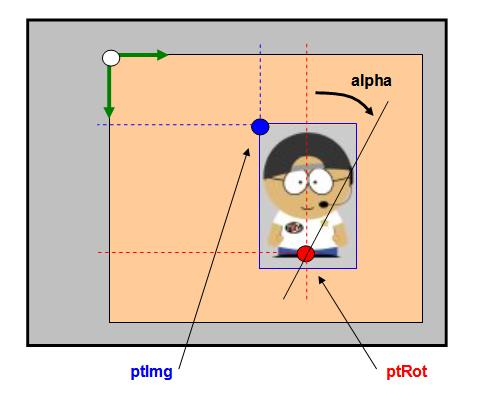News:

2 UCanCode Advance E-XD++ HMI & SCADA Source Code Solution for C/C++, .NET V2023 is released!

3 UCanCode Advance E-XD++ GIS SVG Drawing and Printing Solution Source Code Solution for C/C++, .NET V2023 is released!